Math worksheetsCustom math worksheets at your fingertips

# Our Top Ten lists

Just in case you are curious, to give you some inspiration, or just for browsing: Here are our Top Ten lists. These lists are created daily from current actual activity of our users.

Look at:

Join the crowd, register here.

## Top Ton most popular math problems

These are the problems that are used most to build customized worksheets.

Click the Quickname to go to the detail view page for the problem. You can download sample worksheets and solution sheets for this problem there, too. If you remember the problem's Quickname, you can add it to your own worksheet quickly: in the bottom right corner of the worksheet editor, there is a form field for it.

PlaceProblem's nameDescriptionQuicknameExample
1.Factorisation of termsFactorise a term with variables82312.Add sub split one digit numberAddition and subtraction by splitting one digit number4896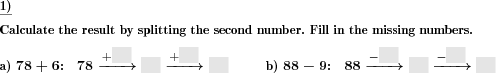3.Add numbersNumbers with or without decimal places have to be added.4897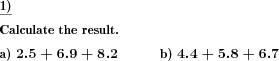4.Columnar subtractionColumnar addition of multiple subtrahends.9777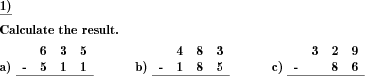5.Decimal fraction div by power of 10A decimal fraction has to be divided by a power of ten.86006.Decimal fraction multiply by power of 10A decimal fraction has to be multiplied by a power of ten.8565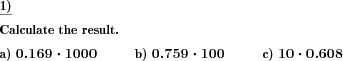7.Number wall sums of whole numbersIn the number wall missing sums have to be added.8704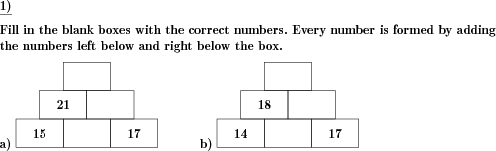8.Percent rate calculation from base and percentageBase value and percentage are given, calculate the rate and fill in the empty box.1902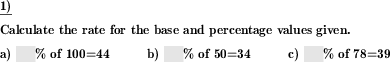9.Columnar addition with blanksBlanks in columnar addition to be filled in correctly.1442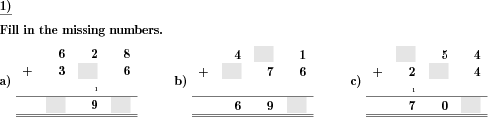10.Convert to like fractionsConvert multiple fractions to like quantities6760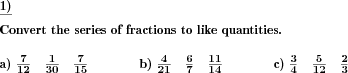## The top ten most frequently generated problems

Here at dw-math, math problems are generated automatically according to your needs. This is why all problems look different. These are the problems that our users have been re-generating the most during the last 60 days. This means that there are problems have been added to a worksheet or have been refreshed on existing worksheets frequently..

Click the Quickname to go to the detail view page for the problem. You can download sample worksheets and solution sheets for this problem there, too. If you remember the problem's Quickname, you can add it to your own worksheet quickly: in the bottom right corner of the worksheet editor, there is a form field for it.

PlaceProblem's nameDescriptionQuicknameExample
1.Factorisation of termsFactorise a term with variables82312.Add sub split one digit numberAddition and subtraction by splitting one digit number48963.Add numbersNumbers with or without decimal places have to be added.48974.Columnar subtractionColumnar addition of multiple subtrahends.97775.Decimal fraction div by power of 10A decimal fraction has to be divided by a power of ten.86006.Decimal fraction multiply by power of 10A decimal fraction has to be multiplied by a power of ten.85657.Number wall sums of whole numbersIn the number wall missing sums have to be added.87048.Percent rate calculation from base and percentageBase value and percentage are given, calculate the rate and fill in the empty box.19029.Columnar addition with blanksBlanks in columnar addition to be filled in correctly.144210.Convert to like fractionsConvert multiple fractions to like quantities6760These informational pages with samples describe math problems that can be combined on custom math worksheets with solutions for home and school use.